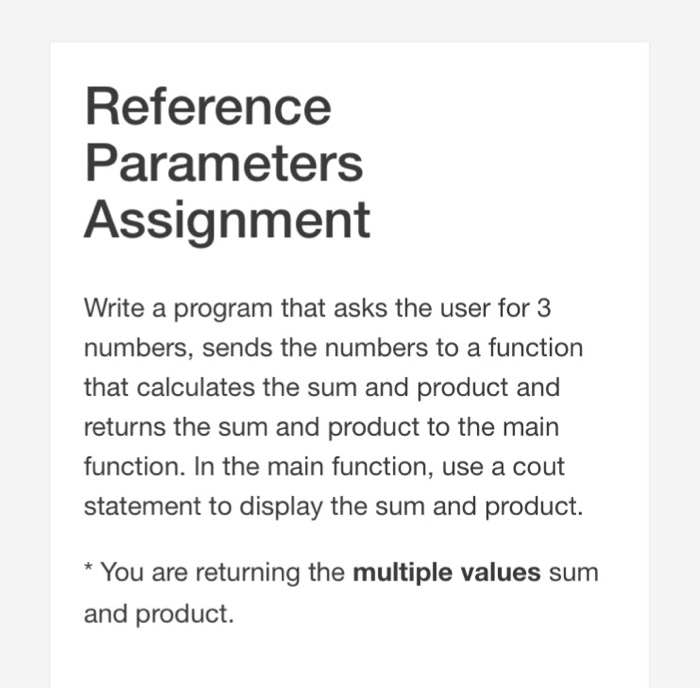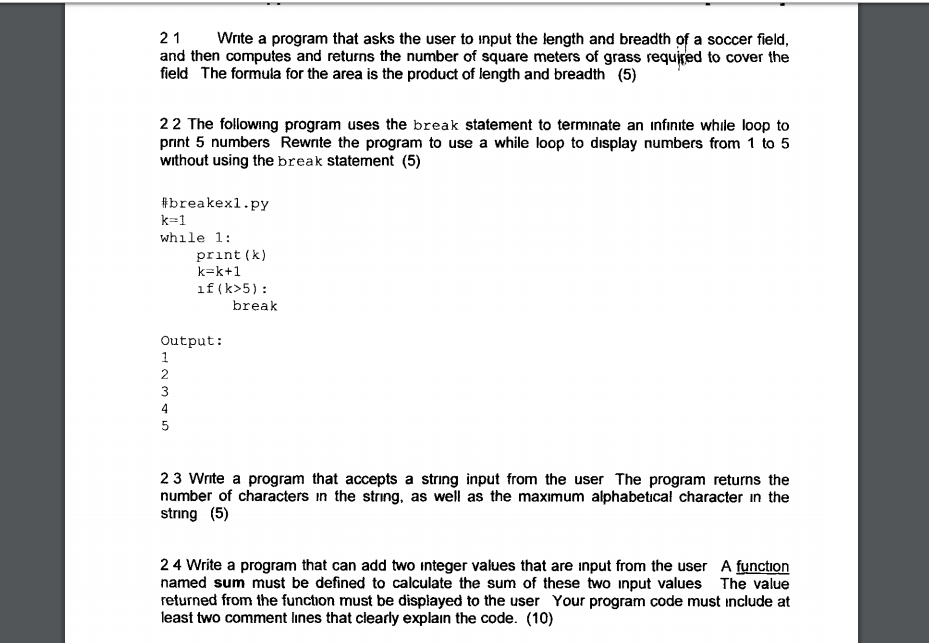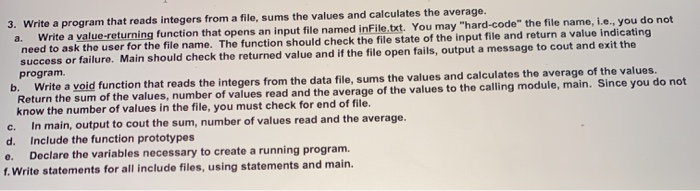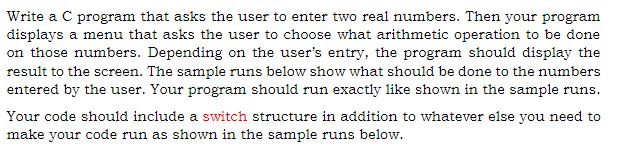Question
Using C++#include <iostream>
using namespace std;

struct Return_value        //Constructing a structure to store sum and product.
{
int sum;
int product;
};

Return_value function(int a,int b,int c)     //Function that will calculate sum and product and return multiple values.
{
int sum=a+b+c;
int product=a*b*c;
return {sum,product};
}

int main()
{   int a,b,c;
cout<<"Enter three numbers"<<endl;
cin>>a>>b>>c; //taking inputs
struct Return_value print= function(a,b,c);
cout<<"sum: "<<print.sum<<endl<<"product: "<<print.product<<endl; //printing the sum and product.
return 0;
}

#### Earn Coins

Coins can be redeemed for fabulous gifts.

Similar Homework Help Questions
• ### 21 Write a program that asks the user to input the length and breadth of a...21 Write a program that asks the user to input the length and breadth of a soccer field, and then computes and returns the number of square meters of grass required to cover the field The formula for the area is the product of length and breadth (5) 22 The following program uses the break statement to terminate an infinite while loop to print 5 numbers Rewrite the program to use a while loop to display numbers from 1 to...

• ### In java, write a program that gets 10 integer numbers from the user using user input,...

In java, write a program that gets 10 integer numbers from the user using user input, and then calculates and display the sum of the numbers that have been read.   Program Requirements: Write the program in three versions with three loops. Put all three loops in the main method of your source code. version1:  use a while loop. version2:  use a do-while loop. version 3:  use a for loop. For each version, use a loop to input 10 int numbers from the user...

• ### C++ 3. Write a program that reads integers from a file, sums the values and calculates...C++ 3. Write a program that reads integers from a file, sums the values and calculates the average. a. Write a value-returning function that opens an input file named in File txt. You may "hard-code" the file name, i.e., you do not need to ask the user for the file name. The function should check the file state of the input file and return a value indicating success or failure. Main should check the returned value and if the file...

• ### Write a driver C++ program that will test the following functions in the same order they...

Write a driver C++ program that will test the following functions in the same order they are written next. You can test your program by reading the following values: n1= 10 and n2= 20 from the user: The main program sends the values of the variables n1 and n2 to the function. The function calculates the average and sends it back to main program through the parameter list as call by reference parameter and will not use the return statement.

• ### You are to write a program IN C++ that asks the user to enter an item's...

You are to write a program IN C++ that asks the user to enter an item's wholesale cost and its markup percentage. It should then display the item's retail price. For example: if the an item's wholesale cost is 5.00 and its markup percentage is 100%, then the item's retail price is 10.00 If an item's wholesale cost is 5.00 and its markup percentage is 50%, then the item's retail price is 7.50 Program design specs. You should have 5...

• ### in c++ language 1.Re-write the following for loop statement by using a while loop statement: int...

in c++ language 1.Re-write the following for loop statement by using a while loop statement: int sum = 0; for(int i=0;i<=1000;i++){                 sum+=i; } 1 (b). Following is the main () function of a program. The program asks a user to enter 150 integers and print the largest integer user entered. int main() {    int score, highest;             //missing portion of the program             return 0;    } 1(c). Find the missing portion of the following code, so the...

• ### Design a program in pseudocode that asks the user to enter a string containing a series...

Design a program in pseudocode that asks the user to enter a string containing a series of ten single-digit numbers with nothing separating them. The program should display the sum of all the single digit numbers in the string except for the highest value digit. You may only use one main module and one sorting function; otherwise, do not modularize your program. For example, if the user enters 5612286470, the sum would be 33. (0+1+2+2+4+5+6+6+7) If there are multiple highest...

• ### Write a C Program that asks the user to input a string and then the user...

Write a C Program that asks the user to input a string and then the user is prompted back whether the input string is a “Palindrome Word” or not. (A Palindrome Word reads the same backward or forward, eg. madam, racecar, etc.) Your program should contain a function that accepts the input string from the main function and returns a value indicating whether the input string is a Palindrome or not. Use Pointers to traverse the string.

• ### Write a C program that asks the user to enter two real numbers. Then your program...Write a C program that asks the user to enter two real numbers. Then your program displays a menu that asks the user to choose what arithmetic operation to be done on those numbers. Depending on the user's entry, the program should display the result to the screen. The sample runs below show what should be done to the numbers entered by the user. Your program should run exactly like shown in the sample runs. make your code run as...

• ### You must write a C program that prompts the user for two numbers (no command line...

You must write a C program that prompts the user for two numbers (no command line input) and multiplies them together using “a la russe” multiplication. The program must display your banner logo as part of a prompt to the user. The valid range of values is 0 to 6000. You may assume that the user will always enter numerical decimal format values. Your program should check this numerical range (including checking for negative numbers) and reprompt the user for...